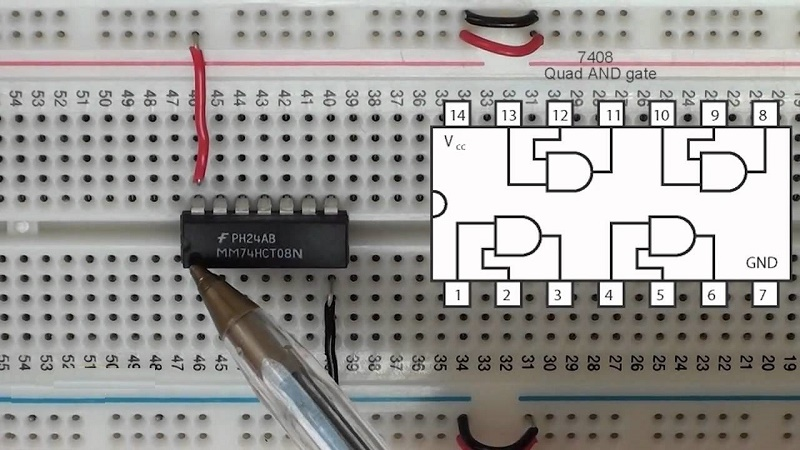Digital Logic Circuits - EE8351, EE6301

Online Study Material, Lecturing Notes, Assignment, Reference, Wiki and important questions and answersNUMBER SYSTEMS AND DIGITAL LOGIC FAMILIES

=> Review of Number Systems
=> Boolean Arithmetic
=> Theorems of Boolean algebra
=> Demorgan's Theorem
=> Canonical Form of Boolean Expressions
=> Min Terms and Max Terms
=> Error Detection and Correction
=> Register Transfer Level ( RTL)
=> TTL
=> DTL Basic Gates
=> Emitter coupled logic (ECL)
=> Important Short Questions and Answers: Digital Logic Circuits: Number Systems and Digital Logic Families

COMBINATIONAL CIRCUITS

=> Minimization Technique
=> Karnaugh Map Method
=> Different Styles of row and column identification - K map
=> Combinational Logic Design
=> Logic Implementation and circuit diagram of Half and Full Adder
=> Half Subtractor
=> Full Subtractor
=> Multipliers
=> Multiplexers
=> Demultiplexers
=> Decoders and Encoders
=> Magnitude Comparator
=> Important Short Questions and Answers: Combinational Circuits

SYNCHRONOUS SEQUENTIAL CIRCUITS

=> RS Flip Flop
=> JK Flip Flop
=> D Flip Flop
=> Analysis and Synthesis of Synchronous Sequential Circuit
=> Design of synchronous sequential circuit
=> Counters and Counters Types
=> Types of shift register
=> Synchronous and Asynchronous Operation
=> Summary of the Types of Flip flop Behaviour
=> Analysis and Design of Sequential Circuits
=> Important Short Questions and Answers: Synchronous Sequential Circuits

ASYNCHRONOUS SEQUENTIAL CIRCUITS AND PROGRAMMABLE LOGIC DEVICES

=> Analysis Procedure of Asynchronous Sequential Circuits
=> Equivalent States and Reduction of State Table
=> Using a One Hot State Assignment
=> Classification of memories
=> ROM Organization
=> Programmable ROM
=> Basic Memory Cell of a PROM: Erasable PROM
=> Random Access Memory
=> VLSI Programmable Logic Devices
=> Programmable Array Logic
=> Fixed Logic Versus Programmable Logic
=> CPLDs and FPGAs
=> The PLD Market
=> Features in common with PALs and FPGAs
=> Important Short Questions and Answers: Asynchronous Sequential Circuits and Programmable Logic Devices

VHDL

=> Hardware Description Language(HDL)
=> Design using HDL
=> HDL and programming languages
=> Combinational logic
=> Sequential logic
=> Clocked sequential system
=> VHDL Operators
=> VHDL for Serial Comparator
=> Code Generation Examples
=> Important Short Questions and Answers: VHDL

EE6301 Digital Logic Circuits - Anna University 2013 Regulation Syllabus - Download Pdf
EE8351 Digital Logic Circuits - Anna University 2017 Regulation Syllabus - Download Pdf

Digital Logic Circuits - EE6301 May June 2015 Question Paper
Digital Logic Circuits - EE6301 May June 2016 Question Paper
Digital Logic Circuits - EE6301 May June 2017 Question Paper
Digital Logic Circuits - EE6301 May June 2018 Question Paper
Digital Logic Circuits - EE6301 Nov Dec 2014 Question Paper
Digital Logic Circuits - EE6301 Nov Dec 2016 Question Paper

Digital Logic Circuits - EE6301 Nov Dec 2017 Question Paper